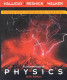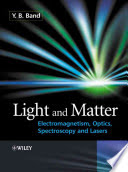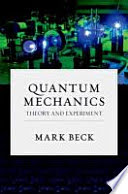### Physics 2R

#### Course Description

Harmonic oscillator, equation of motion, solution, energy considerations. The physical pendulum, linearization of the equation of motion. Damped harmonic oscillation, equation of motion, three kind of solution, energy. Forced oscillation, amplitude and phase, phenomenon of resonance. Transversal waves. Formulating of the wave equation for transversal wave, general solution and harmonic wave. Transmission and reflection of waves on the barrier of two media. Standing waves. Energy and power of the transversal wave. The flux of the electric field. The flux of the magnetic field. The curl of electric and magnetic field. Lorentz force. Maxwell’s equations in vacuum. A plane electromagnetic wave, polarization of the electromagnetic wave. The Poynting’s vector, energy flux density. Laws of the geometrical optics. The principle of the least time. Interference of the coherent sources, Young’s experiment. Diffraction. Polarization. Black body radiation. The photoelectric effect. De Broglie’s relation. Electron diffraction. The Schrödinger equation. Wave function. Free particle. One-dimensional square potential well. Potential barrier. Uncertainty relations.

#### General Competencies

Students completing this course will: understand, appreciate and utilize basics of optics, wave theory and atomic physics in modern technologies and its devices; understand the fundamental principles of physics to prepare students to continue education in modern science and technology, as well as forming a foundation for life-long learning.

#### Learning Outcomes

1. Analyze mechanical oscillatory systems.
2. Apply the linearization technique to equations of motion of the oscillatory systems.
3. Explain the wave equation in non-dispersive medium.
4. Derive the electromagnetic wave equation from the Maxwell’s equations.
5. Explain the phenomena of interference, diffraction and polarization of light.
6. Explain the law of black body radiation by the Planck’s quantum mechanics.
7. Analyze one-dimensional quantum mechanical systems.

#### Forms of Teaching

Lectures

Lectures are delivered to groups of approximately 120 students using electronic presentations, detailed derivations on the blackboard and demonstration experiments.

Exams

Written mid-term exam and the final exam consist of four excercises and a number of multiple choice questions.

Laboratory Work

Students perform six laboratory experiments, carry out the analisys of the measured data and write the final report for each experiment.

Experiments

Lectures are supported by demonstration experiments that illustrate the concepts of physics. Approximately 30 mins / week.

Consultations

At least once a week each professor is available to the students for consultations.

E-learning

During the semester homework assignments are delivered to the students through the e-learning system Merlin (Moodle).

Other Forms of Group and Self Study

In approximately 4 terms per semester additional exercise-solving skills are demonstrated by assistants.

Continuous Assessment Exam
Type Threshold Percent of Grade Threshold Percent of Grade
Laboratory Exercises 5 % 10 % 5 % 10 %
Homeworks 0 % 10 % 0 % 10 %
Mid Term Exam: Written 0 % 40 % 0 %
Final Exam: Written 0 % 40 %
Exam: Written 0 % 80 %
##### Comment:

In the mid-term exam and in the final exam one (of four) excercises must be correctly completed. In the written exam two (of six) excercises must be correctly completed.

#### Week by Week Schedule

1. Simple harmonic oscillations
2. Damped harmonic and forced oscillations
3. Mechanical waves I
4. Mechanical waves II
5. Electromagnetism: Introduction
6. Maxwell’s equations
7. Electromagnetic waves
8. MIDTERM EXAM
9. Geometrical optics
10. Wave optics
11. Introduction to modern physics
12. The structure of the atom
13. Introduction to quantum mechanical waves
14. Introduction to quantum mechanical waves II
15. FINAL EXAM

#### Study Programmes

[FER2-HR] Computing - study
Elective course from a group of mandatory courses-Physics 2 (3. semester)

#### Literature

V.Henč-Bartolić, P.Kulišić (2004.), Valovi i optika, Školska kniga, Zagreb
D. Horvat (2011.), Fizika II - Titranje, valovi, elektromagnetizam, optika i uvod u modernu fiziku, Neodidakta, Zagreb
V. Henč-Bartolić, M. Baće. P. Kulišić, L. Bistričić, D. Horvat, Z. Narančić, T. Petković, D. Pevec (2002.), Riješeni zadaci iz valova i optike, Školska knjiga, ZagrebD. Halliday, R. Resnick, J. Walker (2014.), Fundamentals of Physics D. Halliday, R. Resnick, J. Walker J. Wiley, New York 1993, J. Wiley, New YorkY. B. Band (2006.), Light and Matter: Electromagnetism, Optics, Spectroscopy and Laser, J. Wiley and Sons, New YorkM. Beck (2012.), Quantum Mechanics: Theory and Experiment, Oxford University Press

#### General

ID 141452
Winter semester
5 ECTS
L1 English Level
L2 e-Learning
60 Lectures
0 Seminar
0 Exercises
15 Laboratory exercises
0 Project laboratory